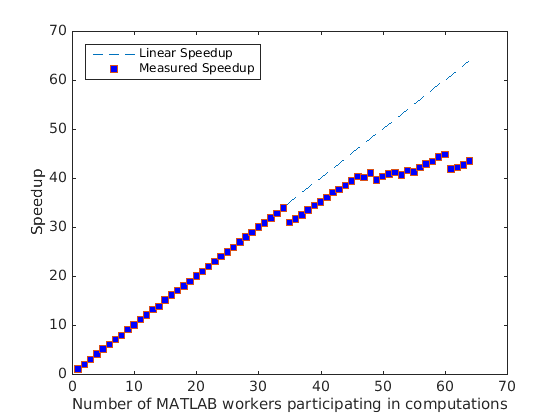# ブラックジャックを使用した parfor のシンプルなベンチマーク

この例では、カード ゲームのブラックジャック (「21」とも呼ばれます) を繰り返しプレイすることによって `parfor` 構造のベンチマークを実行します。`parfor` を使用して、MATLAB® ワーカーの数を変化させながら、このカード ゲームを複数回並列でプレイします。ただし、常に同じプレーヤー数とゲーム数を使用するものとします。

### 並列バージョン

```for i = 1:numPlayers S(:, i) = playBlackjack(); end ```

これを以下の同等な `parfor` ループに変換します。

```parfor i = 1:numPlayers S(:, i) = playBlackjack(); end ```

これをわずかに変更します。具体的には、`parfor` のオプション引数を指定して、計算に使用するワーカー数を `n` に制限するように指示します。実際のコードは以下のようになります。

```dbtype pctdemo_aux_parforbench ```
```1 function S = pctdemo_aux_parforbench(numHands, numPlayers, n) 2 %PCTDEMO_AUX_PARFORBENCH Use parfor to play blackjack. 3 % S = pctdemo_aux_parforbench(numHands, numPlayers, n) plays 4 % numHands hands of blackjack numPlayers times, and uses no 5 % more than n MATLAB(R) workers for the computations. 6 7 % Copyright 2007-2009 The MathWorks, Inc. 8 9 S = zeros(numHands, numPlayers); 10 parfor (i = 1:numPlayers, n) 11 S(:, i) = pctdemo_task_blackjack(numHands, 1); 12 end ```

### 並列プールのステータスの確認

```p = gcp; if isempty(p) error('pctexample:backslashbench:poolClosed', ... ['This example requires a parallel pool. ' ... 'Manually start a pool using the parpool command or set ' ... 'your parallel preferences to automatically start a pool.']); end poolSize = p.NumWorkers; ```

### ベンチマークの実行: ウィーク スケーリング

2 ～ `poolSize` 個のワーカーを使用して、ベンチマーク計算の実行時間を測定します。ここでは、ウィーク スケーリングを使用します。つまり、問題の規模をワーカーの数に応じて大きくするということです。

```numHands = 2000; numPlayers = 6; fprintf('Simulating each player playing %d hands.\n', numHands); t1 = zeros(1, poolSize); for n = 2:poolSize tic; pctdemo_aux_parforbench(numHands, n*numPlayers, n); t1(n) = toc; fprintf('%d workers simulated %d players in %3.2f seconds.\n', ... n, n*numPlayers, t1(n)); end ```
```Simulating each player playing 2000 hands. 2 workers simulated 12 players in 10.81 seconds. 3 workers simulated 18 players in 10.67 seconds. 4 workers simulated 24 players in 10.57 seconds. 5 workers simulated 30 players in 10.57 seconds. 6 workers simulated 36 players in 10.71 seconds. 7 workers simulated 42 players in 10.63 seconds. 8 workers simulated 48 players in 10.87 seconds. 9 workers simulated 54 players in 10.54 seconds. 10 workers simulated 60 players in 10.73 seconds. 11 workers simulated 66 players in 10.58 seconds. 12 workers simulated 72 players in 10.68 seconds. 13 workers simulated 78 players in 10.56 seconds. 14 workers simulated 84 players in 10.89 seconds. 15 workers simulated 90 players in 10.62 seconds. 16 workers simulated 96 players in 10.63 seconds. 17 workers simulated 102 players in 10.70 seconds. 18 workers simulated 108 players in 10.70 seconds. 19 workers simulated 114 players in 10.79 seconds. 20 workers simulated 120 players in 10.72 seconds. 21 workers simulated 126 players in 10.74 seconds. 22 workers simulated 132 players in 10.75 seconds. 23 workers simulated 138 players in 10.74 seconds. 24 workers simulated 144 players in 10.72 seconds. 25 workers simulated 150 players in 10.74 seconds. 26 workers simulated 156 players in 10.76 seconds. 27 workers simulated 162 players in 10.74 seconds. 28 workers simulated 168 players in 10.72 seconds. 29 workers simulated 174 players in 10.76 seconds. 30 workers simulated 180 players in 10.69 seconds. 31 workers simulated 186 players in 10.76 seconds. 32 workers simulated 192 players in 10.76 seconds. 33 workers simulated 198 players in 10.79 seconds. 34 workers simulated 204 players in 10.74 seconds. 35 workers simulated 210 players in 12.12 seconds. 36 workers simulated 216 players in 12.19 seconds. 37 workers simulated 222 players in 12.19 seconds. 38 workers simulated 228 players in 12.14 seconds. 39 workers simulated 234 players in 12.15 seconds. 40 workers simulated 240 players in 12.18 seconds. 41 workers simulated 246 players in 12.18 seconds. 42 workers simulated 252 players in 12.14 seconds. 43 workers simulated 258 players in 12.24 seconds. 44 workers simulated 264 players in 12.25 seconds. 45 workers simulated 270 players in 12.23 seconds. 46 workers simulated 276 players in 12.23 seconds. 47 workers simulated 282 players in 12.55 seconds. 48 workers simulated 288 players in 12.52 seconds. 49 workers simulated 294 players in 13.24 seconds. 50 workers simulated 300 players in 13.28 seconds. 51 workers simulated 306 players in 13.36 seconds. 52 workers simulated 312 players in 13.53 seconds. 53 workers simulated 318 players in 13.98 seconds. 54 workers simulated 324 players in 13.90 seconds. 55 workers simulated 330 players in 14.29 seconds. 56 workers simulated 336 players in 14.23 seconds. 57 workers simulated 342 players in 14.25 seconds. 58 workers simulated 348 players in 14.32 seconds. 59 workers simulated 354 players in 14.26 seconds. 60 workers simulated 360 players in 14.34 seconds. 61 workers simulated 366 players in 15.60 seconds. 62 workers simulated 372 players in 15.75 seconds. 63 workers simulated 378 players in 15.79 seconds. 64 workers simulated 384 players in 15.76 seconds. ```

これを、MATLAB® で標準的な `for` ループを使用した場合の実行時間と比較します。

```tic; S = zeros(numHands, numPlayers); for i = 1:numPlayers S(:, i) = pctdemo_task_blackjack(numHands, 1); end t1(1) = toc; fprintf('Ran in %3.2f seconds using a sequential for-loop.\n', t1(1)); ```
```Ran in 10.70 seconds using a sequential for-loop. ```

### 高速化のプロット

```speedup = (1:poolSize).*t1(1)./t1; fig = pctdemo_setup_blackjack(1.0); fig.Visible = 'on'; ax = axes('parent', fig); x = plot(ax, 1:poolSize, 1:poolSize, '--', ... 1:poolSize, speedup, 's', 'MarkerFaceColor', 'b'); t = ax.XTick; t(t ~= round(t)) = []; % Remove all non-integer x-axis ticks. ax.XTick = t; legend(x, 'Linear Speedup', 'Measured Speedup', 'Location', 'NorthWest'); xlabel(ax, 'Number of MATLAB workers participating in computations'); ylabel(ax, 'Speedup'); ```### 高速化の分布の測定

```numIter = 100; t2 = zeros(1, numIter); for i = 1:numIter tic; pctdemo_aux_parforbench(numHands, poolSize*numPlayers, poolSize); t2(i) = toc; if mod(i,20) == 0 fprintf('Benchmark has run %d out of %d times.\n',i,numIter); end end ```
```Benchmark has run 20 out of 100 times. Benchmark has run 40 out of 100 times. Benchmark has run 60 out of 100 times. Benchmark has run 80 out of 100 times. Benchmark has run 100 out of 100 times. ```

### 高速化の分布のプロット

```speedup = t1(1)./t2*poolSize; clf(fig); ax = axes('parent', fig); hist(speedup, 5); a = axis(ax); a(4) = 5*ceil(a(4)/5); % Round y-axis to nearest multiple of 5. axis(ax, a) xlabel(ax, 'Speedup'); ylabel(ax, 'Frequency'); title(ax, sprintf('Speedup of parfor with %d workers', poolSize)); m = median(speedup); fprintf(['Median speedup is %3.2f, which corresponds to '... 'efficiency of %3.2f.\n'], m, m/poolSize); ```
```Median speedup is 43.37, which corresponds to efficiency of 0.68. ```